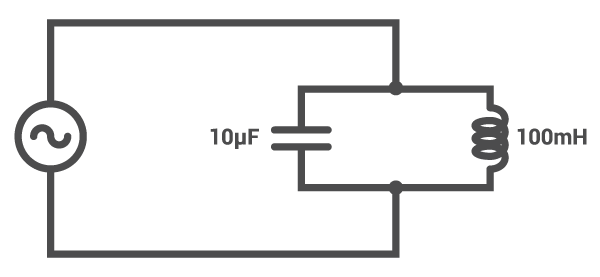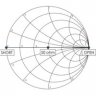# LC Tank Circuit Resonance Calculator

## This calculator computes the resonant frequency of a parallel inductor and capacitor "tank" circuit.

(MHz)

### What is a Tank Circuit?

A tank circuit is a parallel combination of a capacitor and inductor and is the most common "resonant" circuit. When operating at the resonant frequency, an LC tank circuit absorbs maximum power. This tool is designed to calculate the resonant frequency of a tank circuit if the capacitance and inductance values are known.### Calculating the Resonant Frequency of a Tank Circuit

$$f_{r} = \frac{1}{2\pi \sqrt{LC}}$$

Where:

$$f_{r}$$ = resonant frequency (Hz)

$$L$$ = circuit inductance (H)

$$C$$ = circuit capacitance (F)

### Applications

The resonance of tank circuits has many important applications in electrical engineering, particularly in radio technology. For instance, without tank circuits, there would be no radio transmitters or receivers. The simplest radio transmitter involves the use of a class C amplifier with a tank circuit at its load side. When this circuit is powered, the tank circuit generates enough energy to couple the signal from the amplifier to the antenna, and thus the signal radiates to space. Similarly, a radio receiver employs a tank circuit when receiving a signal, by tuning it to the frequency of that signal.

Textbook - Tank Circuit Resonance

Textbook - Resonant Filters

Worksheet - Resonance•chris clement March 04, 2021

Many values of L and C can resonate at the same frequency probably in a hyperbola due to the inverse relationship. Where is the “best” pair of values?

Like.
•R.J. December 05, 2021
Depends on the application but typically if you match the impedance of the the parallel LC to the source impedance that's a good starting point. Your circuit will have maximum power transfer in that configuration. Also be mindful of standard cap and inductor values.
Like.
• Z
ziggy90127 November 19, 2021

I’ve setup a tank circuit with a known inductor, and an unknown capacitor, and measured the resonant frequency with an oscilloscope. It would be nice to have a calculator to solve for C.  Likewise with an unknown L.

Like.